## A non-trivial circular argument can often be usefully perturbed to a non-circular one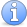Incomplete This article is incomplete. This article is not a stub, but needs more concrete and interesting examples.

### Quick description

One of the first things we learn in mathematical logic is that circular arguments are invalid; if a proof of a statement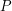uses that statement(either explicitly or implicitly) as one of the hypotheses, then no conclusion can be drawn. And, indeed, if circular reasoning was allowed, then one could easily "prove" any statement one wished, since any statementcan be deduced from itself in a trivial fashion.

However, suppose one was able to obtain a non-trivial deduction of the form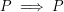: one where the hypothesisis somehow combined with some other deep facts in order to return, in a somewhat unexpected manner, back toagain (or something very close to). Then, in many cases, one can convert this circular (and hence invalid) logical argument into a rigorous one that can actually be used to deriveunconditionally, provided that one can also verify some sort of "base case", and provided one has some sort of "continuity". Here are some general instances of this idea:

• Suppose one has a statement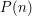that depends on a natural number parameter. If one takesas a hypothesis and then deducesas a conclusion, nothing useful can be drawn from this; but if instead one takesas a hypothesis and somehow manages to deduce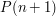as a conclusion, then (providing one can also verify a base case such as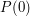or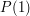), one can now use mathematical induction to rigorously and unconditionally concludefor all.

• A well-known variant is the principle of strong induction: if, for each, one can deduceassuming that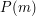is true for all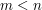, then this is not a circular deduction, but is in fact strong enough to establishfor all(provided thatranges in a well-ordered set, such as the natural numbers).

• Suppose one has a continuous function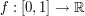, and one wishes to establish the bound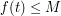for all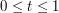and some given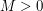. If one was only able to establish the boundat eachassuming the bound, then no conclusion can be drawn. If however, one was able to establish the boundassuming the bound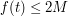at that value of, then (assuming one can also verify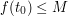for at least one "base" value of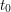) one can show thatfor allunconditionally, since if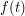exceeded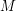anywhere then by the intermediate value theorem we would have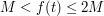for at least one value of, a contradiction. (Alternatively, one can argue that the set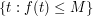is open, closed, and non-empty, and thus must equal all of the connected interval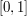). Clearly one can replaceby any other connected set here.

• There is also a "strong induction" version of the previous trick: if, for each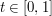one can proveassuming that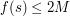for all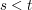, thenin fact holds for all. Again, one can replaceby other connected intervals (though for intervals containing, one needs a base case to get started).

• There is nothing special about the factor ofhere. Even an epsilon gain over the trivial implication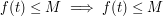, say to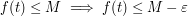, makes all the difference between a useless implication and a highly useful one.

• If one wants to show some sort of "exact" statement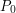, and one has discovered some sort of "non-trivial" implication of the form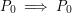, then no conclusion can initially be drawn; but if however this non-trivial implication can be perturbed to something like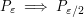for all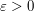, where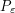is some "approximate" version of, and one can already show that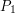(say) is true, then this implies thatis true for arbitrarily small, which can sometimes be used to recover the original statement(cf.

• Again, there is nothing special about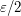in the previous scheme: any implication of the form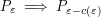would work, so long as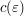is bounded away from zero wheneveris bounded away from zero.

This type of idea is particularly common in nonlinear PDE, where it goes by such names as the "bootstrap argument", "the continuity method", and "the method of a priori estimates": if one wants to achieve some task, such as construct a solution to a given PDE problem with some bounds, assume that such a solution exists already, and use the assumed bounds to deduce slightly better bounds on the solution. In many cases, such an "a priori estimate" can be bootstrapped (by exploiting some continuity properties of the solution or the PDE) into a rigorous and unconditional existence argument.

### Prerequisites

Combinatorics, partial differential equations

### Example 1

(Stability of a particle in a potential well; more suggestions welcome)

### Example 2

(Density increment arguments and energy increment arguments. Once again, Roth's theorem is the poster child...)